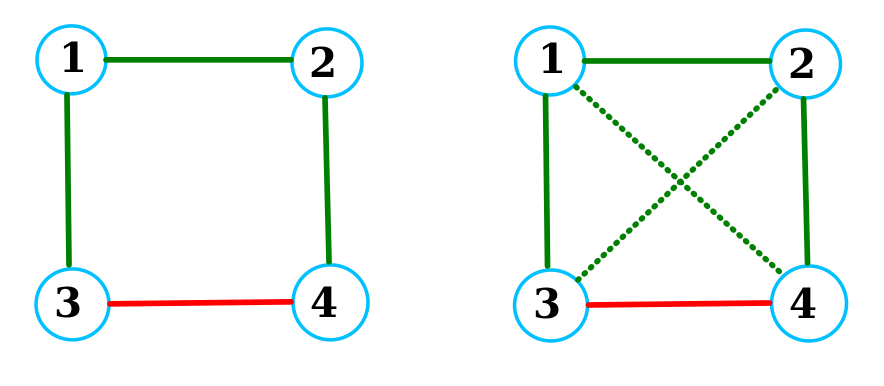# Programming contests

## DEIK Regionális Programozó Csapatverseny, egyetemi kategória, 2022. december 4.

December 4, 2022 10:00 AM – December 4, 2022 3:15 PM

# future friendsIn a group the relation between the members can be either friendly or neutral or hostile. (the relations are symmetric!) It is possible, that between some members the currently neutral relation will become friendly. According to some scientists, if A and B are in neutral relation, but one can get from A to B through a sequence of friendly relations, then the relation of A and B will become friendly in the future, for we will refer to A and B as a future friends.

If in a group of four people, the pairs `(1,2), (1,3), (1,4)` friends and the `(3,4)` is in hostile relation, then the left part of the figure above depicts the current situation. Green and red edges are used for the friendly and hostile relations, respectively. The future friends are in the right part of the figure connected by dotted-green edges:

```    1 and 4 -> are in neutral relation, but they are connected by a 1 -> 2 -> 4 friendly path
2 and 3 -> are in neutral relation, but they are connected by a 2 -> 1 -> 3 friendly path
3 and 4 are also connected by a 2 -> 1 -> 3 friendly path, but they are in a hostile relation
```
One can conclude, that in this group each member has exactly `1` future friend, therefore the group's average number of future friends is `1=(1+1+1+1)/4`.

Task: Given the relations in a group compute the average number of future friends.

## Input Specification

``````
N B E
x1 y1
...
xB yB
u1 v1
...
uE vE
```
```

The three space separated numbers `1 ≤ N,B,E ≤ 104` in the first line describe the number of people, friendly and hostile relations in the group respectively. In each of the next `B` lines there are two space separated numbers describing a friendly relation. In each the last `E` lines of the input there are two space separated numbers describing a hostile relation. Each of the relation is described by a pair of integers `x,y`, where `x≠y` and `1 ≤ x,y ≤ 10`. No relation occurs more than once in the input.

## Output Specification

```M
```

A single line with the average number of future friends for the group described in the input. Round your result to `6` decimal places.

## Sample Input 1

1. `4 3 1`
2. `1 2`
3. `3 1`
4. `2 4`
5. `3 4`

## Sample Output 1

1. `1.000000`

## Sample Input 2

1. `2 0 0`

## Sample Output 2

1. `0.000000`

## Sample Input 3

1. `4 4 1`
2. `2 1`
3. `1 3`
4. `3 2`
5. `3 4`
6. `4 1`
1. `0.500000`